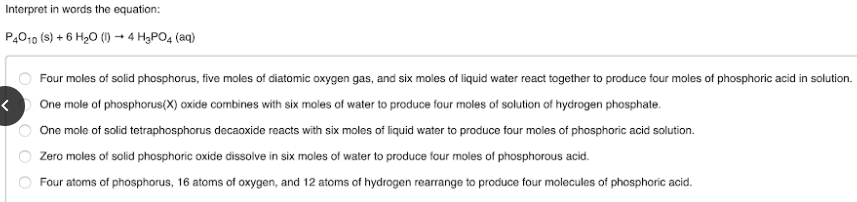# Interpret in words the equation: P4O10 (s) + 6 H2O (l) → 4 H3PO4 (aq) a. Four moles of solid phosphorus, five moles of diatomic oxygen gas, and six moles of liquid water react together to produce four moles of phosphoric acid in solution. b. One mole of phosphorus (x) oxide combines with six moles of water to produce four moles of solution of hydrogen phosphate. c. One mole of solid tetraphosphorus decaoxide reacts with six moles of liquid water to produce four moles of phosphoric acid solution. d. Zero moles of solid phosphoric oxide dissolve in six moles of water to produce four moles of phosphorous acid. e. Four atoms of phosphorus, 16 atoms of oxygen, and 12 atoms of hydrogen rearrange to produce four molecules of phosphoric acid.